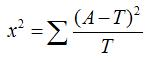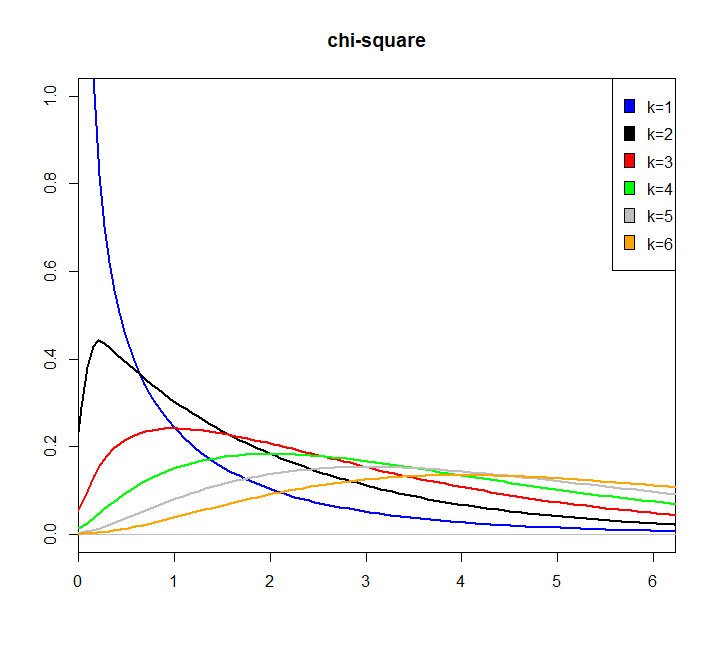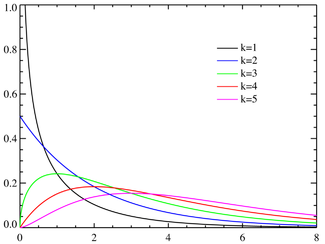感冒人数 未感冒人数 合计 感冒率 喝牛奶组 43 96 139 30.94% 不喝牛奶组 28 84 112 25.00% 合计 71 180 251 28.29%

 感冒人数 未感冒人数 合计 喝牛奶组 =139*0.2829 =139*(1-0.2829) 139 不喝牛奶组 =112*0.2829 =112*(1-0.2829) 112

 感冒人数 未感冒人数 合计 喝牛奶组 39.3231 99.6769 139 不喝牛奶组 31.6848 80.3152 112 合计 71 180 251x2用于衡量实际值与理论值的差异程度（也就是卡方检验的核心思想），包含了以下两个信息：
1. 实际值与理论值偏差的绝对大小（由于平方的存在，差异是被放大的）
2. 差异程度与理论值的相对大小体重下降 体重未下降 合计 体重下降率 吃晚饭组 123 467 590 20.85% 不吃晚饭组 45 106 151 29.80% 合计 168 573 741 22.67%

1. 建立假设检验：

H0：r1＝r2，不吃晚饭对体重下降没有影响，即吃不吃晚饭的体重下降率相等；
H1：r1≠r2，不吃晚饭对体重下降有显著影响，即吃不吃晚饭的体重下降率不相等。α=0.05

2. 计算理论值

 体重下降 体重未下降 合计 吃晚饭组 133.765 456.234 590 不吃晚饭组 34.2348 116.765 151 合计 168 573 741

3. 计算卡方值4. 查卡方表求P值

R语言实现：

``````x1 = rnorm(1000000)
x2 = rnorm(1000000)
x3 = rnorm(1000000)
x4 = rnorm(1000000)
x5 = rnorm(1000000)
x6 = rnorm(1000000)

Q1 = x1^2
Q2 = x1^2 + x2^2
Q3 = x1^2 + x2^2 + x3^2
Q4 = x1^2 + x2^2 + x3^2 + x4^2
Q5 = x1^2 + x2^2 + x3^2 + x4^2 + x5^2
Q6 = x1^2 + x2^2 + x3^2 + x4^2 + x5^2 + x6^2

par(mfrow=c(1,1))
plot(density(Q1),xlim=c(0.23,6),ylim = c(0,1),breaks = 200,col = 'blue',lwd=2,main='chi-square',xlab = '',ylab='')
lines(density(Q2),col='black',lwd=2)
lines(density(Q3),col='red',lwd=2)
lines(density(Q4),col='green',lwd=2)
lines(density(Q5),col='gray',lwd=2)
lines(density(Q6),col='orange',lwd=2)
legend('topright',c('k=1','k=2','k=3','k=4','k=5','k=6'),fill = c('blue','black','red','green','gray','orange'))``````